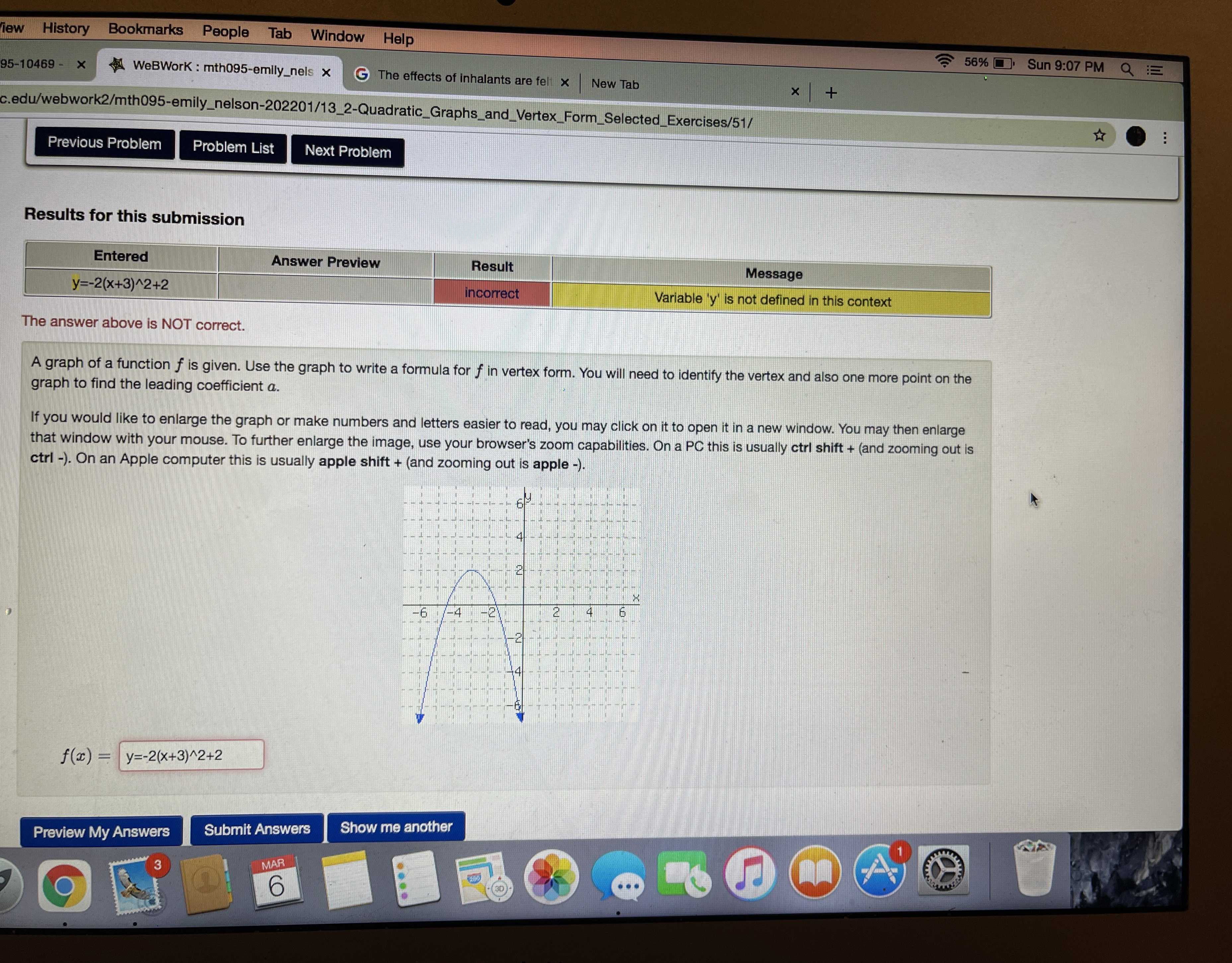### ¿Todavía tienes preguntas de matemáticas?

Pregunte a nuestros tutores expertos
Algebra
PreguntaA graph of a function $$f$$ is given. Use the graph to write a formula for $$f$$ in vertex form. You will need to identify the vertex and also one more point on the graph to find the leading coefficient $$a$$ .

If you would like to enlarge the graph or make numbers and letters easier to read, you may click on it to open it in a new window. You may then enlarge that window with your mouse. To further enlarge the image, use your browser's zoom capabilities. On a PC this is usually ctrl shift + (and zooming out is ctrl -). On an Apple computer this is usually apple shift + (and zooming out is apple -).

f(x)=-x$$^{2}$$-6x-7